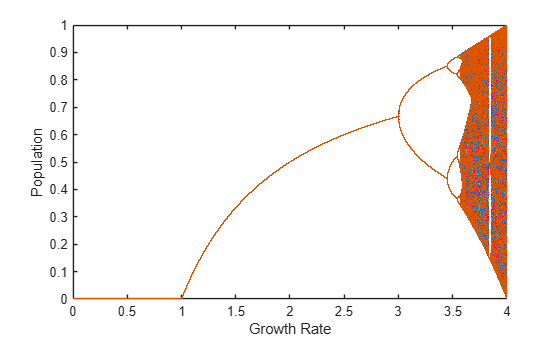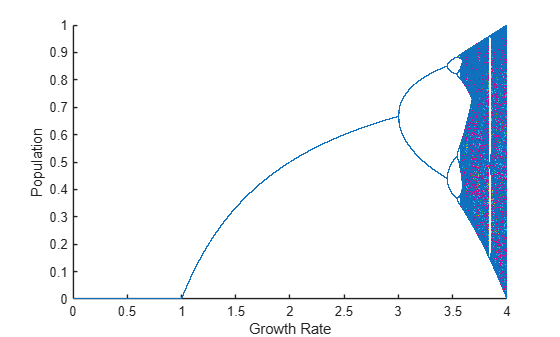Main Content

# Run MATLAB Functions on Multiple GPUs

This example shows how to run MATLAB code on multiple GPUs in parallel, first on your local machine, then scaling up to a cluster. As a sample problem, the example uses the logistic map, an equation that models the growth of a population.

A growing number of features in MATLAB offer automatic parallel support, including multi-gpu support, without requiring any extra coding. For details, see Run MATLAB Functions with Automatic Parallel Support. For example, the `trainNetwork` function offers multi-gpu support for training of neural networks and inference. For more information, see Scale Up Deep Learning in Parallel and in the Cloud (Deep Learning Toolbox).

### Use a Single GPU

To run computations on a single GPU, use `gpuArray` objects as inputs to GPU-enabled MATLAB functions. To learn more about GPU-enabled functions, see Run MATLAB Functions on a GPU.

Create gpuArrays for the growth rate, `r`, and the population, `x`. For more information on creating gpuArrays, see Establish Arrays on a GPU.

```N = 1000; r = gpuArray.linspace(0,4,N); x = rand(1,N,'gpuArray');```

Use a simple algorithm to iterate the logistic map. Because the algorithm uses GPU-enabled operators on gpuArrays, the computations run on the GPU.

```numIterations = 1000; for n=1:numIterations x = r.*x.*(1-x); end```

When the computations are done, plot the growth rate against the population.

`plot(r,x,'.');`If you need more performance, gpuArrays supports several options. For a list, see the `gpuArray` function page. For example, the algorithm in this example only performs element-wise operations on gpuArrays, and so you can use the `arrayfun` function to precompile them for GPU.

### Use Multiple GPUs with `parfor`

You can use `parfor` loops to distribute `for`-loop iterations among parallel workers. If your computations use GPU-enabled functions, then the computations run on the GPU of the worker. For example, if you use the Monte Carlo method to randomly simulate the evolution of populations, simulations are computed with multiple GPUs in parallel using a `parfor` loop.

Create a parallel pool with as many workers as GPUs available. To determine the number of GPUs available, use the `gpuDeviceCount` function. By default, MATLAB assigns a different GPU to each worker for best performance. For more information on selecting GPUs in a parallel pool, see Use Multiple GPUs in Parallel Pool.

```numGPUs = gpuDeviceCount("available"); parpool(numGPUs);```
```Starting parallel pool (parpool) using the 'local' profile ... connected to 2 workers. ```

Define the number of simulations, and create an array in the GPU to store the population vector for each simulation.

```numSimulations = 100; X = zeros(numSimulations,N,'gpuArray');```

Use a `parfor` loop to distribute simulations to workers in the pool. The code inside the loop creates a random gpuArray for the initial population, and iterates the logistic map on it. Because the code uses GPU-enabled operators on gpuArrays, the computations automatically run on the GPU of the worker.

```parfor i = 1:numSimulations X(i,:) = rand(1,N,'gpuArray'); for n=1:numIterations X(i,:) = r.*X(i,:).*(1-X(i,:)); end end```

When the computations are done, plot the results of all simulations. Each color represents a different simulation.

```figure plot(r,X,'.');```If you need greater control over your calculations, you can use more advanced parallel functionality. For example, you can use a `parallel.pool.DataQueue` to send data from the workers during computations. For an example, see Plot During Parameter Sweep with parfor.

If you want to generate a reproducible set of random numbers, you can control the random number generation on the worker GPU. For more information, see Control Random Number Streams on Workers.

### Use Multiple GPUs Asynchronously with `parfeval`

You can use `parfeval` to run computations asynchronously on parallel pool workers. If your computations use GPU-enabled functions, then the computations run on the GPU of the worker. As an example, you run Monte Carlo simulations on multiple GPUs asynchronously.

To hold the results of computations after the workers complete them, use future objects. Preallocate an array of future objects for the result of each simulation.

`f(numSimulations) = parallel.FevalFuture;`

To run computations with `parfeval`, you must place them inside a function. For example, `myParallelFcn` contains the code of a single simulation.

`type myParallelFcn`
```function x = myParallelFcn(r) N = 1000; x = gpuArray.rand(1,N); numIterations = 1000; for n=1:numIterations x = r.*x.*(1-x); end end ```

Use a `for` loop to loop over simulations, and use `parfeval` to run them asynchronously on a worker in the parallel pool. `myParallelFcn` uses GPU-enabled functions on gpuArrays, so they run on the GPU of the worker. Because `parfeval` performs the computations asynchronously, it does not block MATLAB, and you can continue working while computations happen.

```for i=1:numSimulations f(i) = parfeval(@myParallelFcn,1,r); end```

To collect the results from `parfeval` when they are ready, you can use `fetchOutputs` or `fetchNext` on the future objects. Also, you can use `afterEach` or `afterAll` to invoke functions on the results automatically when they are ready. For example, to plot the result of each simulation immediately after it completes, use `afterEach` on the future objects. Each color represents a different simulation.

```figure hold on afterEach(f,@(x) plot(r,x,'.'),0);```### Use Multiple GPUs in a Cluster

If you have access to a cluster with multiple GPUs, then you can scale up your computations. Use the `parpool` function to start a parallel pool on the cluster. When you do so, parallel features, such as `parfor` loops or `parfeval`, run on the cluster workers. If your computations use GPU-enabled functions on gpuArrays, then those functions run on the GPU of the cluster worker. To learn more about running parallel features on a cluster, see Scale Up from Desktop to Cluster.

## SupportGet trial now